Home
Hostname: page-component-568f69f84b-h2zp4 Total loading time: 0.244 Render date: 2021-09-17T08:17:42.195Z Has data issue: true Feature Flags: { "shouldUseShareProductTool": true, "shouldUseHypothesis": true, "isUnsiloEnabled": true, "metricsAbstractViews": false, "figures": true, "newCiteModal": false, "newCitedByModal": true, "newEcommerce": true, "newUsageEvents": true }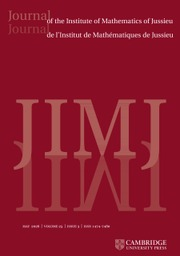Journal of the Institute of Mathematics of Jussieu

# THE RENORMALIZED VOLUME AND UNIFORMIZATION OF CONFORMAL STRUCTURES

Published online by Cambridge University Press:  30 June 2016

## Abstract

We study the renormalized volume of asymptotically hyperbolic Einstein (AHE in short) manifolds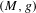$(M,g)$ when the conformal boundary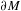$\unicode[STIX]{x2202}M$ has dimension$n$ even. Its definition depends on the choice of metric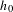$h_{0}$ on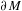$\unicode[STIX]{x2202}M$ in the conformal class at infinity determined by$g$, we denote it by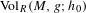$\text{Vol}_{R}(M,g;h_{0})$. We show that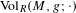$\text{Vol}_{R}(M,g;\cdot )$ is a functional admitting a ‘Polyakov type’ formula in the conformal class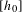$[h_{0}]$ and we describe the critical points as solutions of some non-linear equation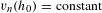$v_{n}(h_{0})=\text{constant}$, satisfied in particular by Einstein metrics. When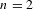$n=2$, choosing extremizers in the conformal class amounts to uniformizing the surface, while if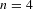$n=4$ this amounts to solving the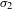$\unicode[STIX]{x1D70E}_{2}$-Yamabe problem. Next, we consider the variation of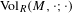$\text{Vol}_{R}(M,\cdot ;\cdot )$ along a curve of AHE metrics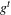$g^{t}$ with boundary metric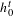$h_{0}^{t}$ and we use this to show that, provided conformal classes can be (locally) parametrized by metrics$h$ solving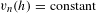$v_{n}(h)=\text{constant}$ and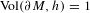$\text{Vol}(\unicode[STIX]{x2202}M,h)=1$, the set of ends of AHE manifolds (up to diffeomorphisms isotopic to the identity) can be viewed as a Lagrangian submanifold in the cotangent space to the space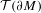${\mathcal{T}}(\unicode[STIX]{x2202}M)$ of conformal structures on$\unicode[STIX]{x2202}M$. We obtain, as a consequence, a higher-dimensional version of McMullen’s quasi-Fuchsian reciprocity. We finally show that conformal classes admitting negatively curved Einstein metrics are local minima for the renormalized volume for a warped product type filling.

## MSC classification

Type
Research Article
Information
Journal of the Institute of Mathematics of Jussieu , September 2018 , pp. 853 - 912

## Access options

Get access to the full version of this content by using one of the access options below. (Log in options will check for institutional or personal access. Content may require purchase if you do not have access.)

## Footnotes

C. G. was partially supported by the A.N.R. project ACG ANR-10-BLAN-0105. S. M. was partially supported by the CNCS project PN-II-RU-TE-2011-3-0053; he thanks the Fondation des Sciences Mathématiques de Paris and the École Normale Supérieure for additional support. J.-M. S. was partially supported by the A.N.R. through projects ETTT, ANR-09-BLAN-0116-01, and ACG, ANR-10-BLAN-0105.

## References

Ahlfors, L. and Bers, L., Riemann’s mapping theorem for variable metrics, Ann. of Math. (2) 72 (1960), 385404.CrossRefGoogle Scholar
Albin, P., Renormalizing curvature integrals on Poincaré–Einstein manifolds, Adv. Math. 221(1) (2009), 140169.CrossRefGoogle Scholar
Anderson, M. T., L 2 curvature and renormalization of AHE metrics on 4-manifolds, Math. Res. Lett. 8 (2001), 171188.CrossRefGoogle Scholar
Bär, C., Gauduchon, P. and Moroianu, A., Generalized cylinders in semi-Riemannian and spin geometry, Math. Z. 249(3) (2005), 545580.CrossRefGoogle Scholar
Bers, L., Simultaneous uniformization, Bull. Amer. Math. Soc. 66 (1960), 9497.CrossRefGoogle Scholar
Besse, A., Einstein Manifolds, Ergebnisse der Mathematik und ihrer Grenzgebiete (3), Volume 10 (Springer, Berlin, 1987).CrossRefGoogle Scholar
Biquard, O., Métriques d’Einstein asymptotiquement symétriques, Astérisque 265 (2000).Google Scholar
Biquard, O., Autodual Einstein versus Kähler–Einstein, Geom. Funct. Anal. 15(3) (2005), 598633.CrossRefGoogle Scholar
Biquard, O., Continuation unique à partir de l’infini conforme pour les métriques d’Einstein, Math. Res. Lett. 15(6) (2008), 10911099.CrossRefGoogle Scholar
Bochner, S., Vector fields and Ricci curvature, Bull. Amer. Math. Soc. 52 (1946), 776797.CrossRefGoogle Scholar
Caffarelli, L., Nirenberg, L. and Spruck, J., The Dirichlet problem for nonlinear second-order elliptic equations. III. Functions of the eigenvalues of the Hessian, Acta Math. 155(3–4) (1985), 261301.CrossRefGoogle Scholar
Chang, S.-Y. A. and Fang, H., A class of variational functionals in conformal geometry, Int. Math. Res. Not. IMRN (7) (2008), Art. ID rnn008.Google Scholar
Chang, S.-Y. A., Fang, H. and Graham, C. R., A note on renormalized volume functionals, Differential Geom. Appl. 33(Suppl) (2014), 246258.CrossRefGoogle Scholar
Chang, S.-Y. A., Gursky, M. J. and Yang, P. C., An equation of Monge–Ampre type in conformal geometry, and four-manifolds of positive Ricci curvature, Ann. of Math. (2) 155(3) (2002), 709787.CrossRefGoogle Scholar
Chang, S.-Y. A., Gursky, M. J. and Yang, P. C., An a priori estimate for a fully nonlinear equation on four-manifolds, J. Anal. Math. 87 (2002), 151186. Dedicated to the memory of Thomas H. Wolff.CrossRefGoogle Scholar
Chang, S.-Y. A., Qing, J. and Yang, P., On the topology of conformally compact Einstein 4-manifolds, in Noncompact Problems at the Intersection of Geometry, Analysis, and Topology, Contemporary Mathematics, Volume 350, pp. 4961 (American Mathematical Society, Providence, RI, 2004).CrossRefGoogle Scholar
Chang, S.-Y. A., Qing, J. and Yang, P. C., On the renormalized volumes for conformally compact Einstein manifolds, in Proceeding Conf. in Geometric Analysis, Moscow 2005.Google Scholar
Chrusciel, P., Delay, E., Lee, J. M. and Skinner, D. N., Boundary regularity of conformally compact Einstein metrics, J. Differential Geom. 69(1) (2005), 111136.CrossRefGoogle Scholar
de Haro, S., Skenderis, K. and Solodukhin, S. N., Holographic reconstruction of space time and renormalization in the AdS/CFT correspondence, Comm. Math. Phys. 217 (2001), 595622.CrossRefGoogle Scholar
Delay, E., TT-eigentensors for the Lichnerowicz Laplacian on some asymptotically hyperbolic manifolds with warped products metrics, Manuscripta Math. 123(2) (2007), 147165.CrossRefGoogle Scholar
Ebin, D. G., The manifold of Riemannian metrics, in Global Analysis (Proc. Sympos. Pure Math., Vol. XV, Berkeley, Calif., 1968), pp. 1140 (American Mathematical Society, Providence, RI, 1970).Google Scholar
Djadli, Z., Guillarmou, C. and Herzlich, M., Opérateurs géométriques, invariants conformes et variétés asymptotiquement hyperboliques, Panoramas et Synthèses, Volume 26 (Société Mathématique de France, Paris, 2008).Google Scholar
Fefferman, C. and Graham, C. R., The Ambient Metric, Annals of Mathematics Studies, Volume 178 (Princeton University Press, Princeton, NJ, 2012), x+113 pp.Google Scholar
Fischer, A. E. and Moncrief, V., The structure of quantum conformal superspace, in Global Structure and Evolution in General Relativity (Karlovassi, 1994), Lecture Notes in Physics, Volume 460, pp. 111173 (Springer, Berlin, 1996).CrossRefGoogle Scholar
Frankel, T., On theorems of Hurwitz and Bochner, J. Math. Mech. 15 (1966), 373377.Google Scholar
Graham, C. R., Volume and area renormalizations for conformally compact Einstein metrics, Rend. Circ. Mat. Palermo Ser. II 63(Suppl) (2000), 3142.Google Scholar
Graham, C. R., Dirichlet-to-Neumann map for Poincaré–Einstein metrics, Announc. Oberwolfach Rep. 2(3) (2005), 22002203.Google Scholar
Graham, C. R., Extended obstruction tensors and renormalized volume coefficients, Adv. Math. 220(6) (2009), 19561985.CrossRefGoogle Scholar
Graham, C. R. and Hirachi, K., The ambient obstruction tensor and Q-curvature, in AdS/CFT Correspondence: Einstein Metrics and their Conformal Boundaries, IRMA Lectures in Mathematics and Theoretical Physics, Volume 8, pp. 5971 (European Mathematical Society, Zürich, 2005).CrossRefGoogle Scholar
Graham, C. R. and Juhl, A., Holographic formula for Q-curvature, Adv. Math. 216(2) (2007), 841853.CrossRefGoogle Scholar
Graham, C. R. and Lee, J., Einstein metrics with prescribed conformal infinity on the ball, Adv. Math. 87 (1991), 186225.CrossRefGoogle Scholar
Graham, C. R. and Zworski, M., Scattering matrix in conformal geometry, Invent. Math. 152(1) (2003), 89118.CrossRefGoogle Scholar
Guillarmou, C., Meromorphic properties of the resolvent on asymptotically hyperbolic manifolds, Duke Math. J. 129(1) (2005), 137.CrossRefGoogle Scholar
Guillarmou, C. and Moroianu, S., Chern-Simons line bundle on Teichmüller space, Geom. Topol. 18(1) (2014), 327377.CrossRefGoogle Scholar
Guan, P., Viaclovsky, J. and Wang, G., Some properties of the Schouten tensor and applications to conformal geometry, Trans. Amer. Math. Soc. 355 (2003), 925933.CrossRefGoogle Scholar
Guillopé, L. and Zworski, M., Upper bounds on the number of resonances for non-compact Riemann surfaces, J. Funct. Anal. 129(2) (1995), 363389.CrossRefGoogle Scholar
Gursky, M. and Viaclovsky, J., Fully nonlinear equations on Riemannian manifolds with negative curvature, Indiana Univ. Math. J. 52(2) (2003), 399419.CrossRefGoogle Scholar
Gursky, M. and Viaclovsky, J., Volume comparison and the k-Yamabe problem, Adv. Math. 187(2) (2004), 447487.CrossRefGoogle Scholar
Hamilton, R. S., The inverse function theorem of Nash and Moser, Bull. Amer. Math. Soc. 7(1) (1982), 65222.CrossRefGoogle Scholar
Henningson, M. and Skenderis, K., The holographic Weyl anomaly, J. High Energy Phys. (7) (1998), Paper 23, 12 pp. (electronic).CrossRefGoogle Scholar
Juhl, A., Families of Conformally Covariant Differential Operators, Q-Curvature and Holography, Progress in Mathematics, Volume 275 (Birkhäuser, 2009).CrossRefGoogle Scholar
Krasnov, K., Holography and Riemann surfaces, Adv. Theor. Math. Phys. 4(4) (2000), 929979.CrossRefGoogle Scholar
Krasnov, K. and Schlenker, J.-M., On the renormalized volume of hyperbolic 3-manifolds, Comm. Math. Phys. 279(3) (2008), 637668.CrossRefGoogle Scholar
Lee, J. M., Fredholm operators and Einstein metrics on conformally compact manifolds, Mem. Amer. Math. Soc. 183(864) (2006).Google Scholar
Marden, A., The geometry of finitely generated kleinian groups, Ann. of Math. (2) 99 (1974), 383462.CrossRefGoogle Scholar
Marden, A., Deformation of Kleinian groups, in Handbook of Teichmüller Theory, Volume I,(ed. Papadopoulos, A.), IRMA Lectures in Mathematics and Theoretical Physics, Volume 11 (European Mathematical Society, 2007). chapter 9.Google Scholar
Matsumoto, Y., A GJMS construction for 2-tensors and the second variation of the total Q-curvature, Pacific J. Math. 262(2) (2013), 437455.CrossRefGoogle Scholar
Mazzeo, R., Elliptic theory of differential edge operators. I, Comm. Partial Differential Equations 16(10) (1991), 16151664.Google Scholar
Melrose, R. B., Calculus of conormal distributions on manifolds with corners, Int. Math. Res. Not. 3 (1992), 5161.CrossRefGoogle Scholar
Melrose, R. B., Manifolds with Corners, Available at http://math.mit.edu/∼rbm/book.html (in preparation).Google Scholar
Mcmullen, C. T., The moduli space of Riemann surfaces is Kähler hyperbolic, Ann. of Math. (2) 151(1) (2000), 327357.CrossRefGoogle Scholar
Obata, M., Certain conditions for a Riemannian manifold to be isometric with a sphere, J. Math. Soc. Japan 14 (1962), 333340.CrossRefGoogle Scholar
Obata, M., The conjectures on conformal transformations of Riemannian manifolds, J. Differential Geometry 6 (1971/72), 247258.CrossRefGoogle Scholar
Osgood, B., Phillips, R. and Sarnak, P., Extremals for determinants of Laplacians, J. Funct. Anal. 80 (1988), 148211.CrossRefGoogle Scholar
Patterson, S. J. and Perry, P., The divisor of Selberg’s zeta function for Kleinian groups (appendix A by Charles Epstein), Duke Math. J. 106 (2001), 321391.Google Scholar
Payne, K. R., Smooth tame Fréchet algebras and Lie groups of pseudodifferential operators, Comm. Pure Appl. Math. 44(3) (1991), 309337.CrossRefGoogle Scholar
Reilly, R. C., On the Hessian of a function and the curvatures of its graph, Michigan Math. J. 20 (1973), 373383.Google Scholar
Rivin, I. and Schlenker, J.-M., The Schläfli formula in Einstein manifolds with boundary, Electron. Res. Announc. Amer. Math. Soc. 5 (1999), 1823.CrossRefGoogle Scholar
Schlenker, J.-M., The renormalized volume and the volume of the convex core of quasifuchsian manifolds, Math. Res. Lett. 20(4) (2013), 773786.CrossRefGoogle Scholar
Sheng, W., Trudinger, N. S. and Wang, X.-J., The Yamabe problem for higher order curvature, J. Differential Geom. 77 (2007), 515553.CrossRefGoogle Scholar
Skenderis, K. and Solodukin, S. N., Quantum effective action from the AdS/CFT correspondence, Phys. Lett. B 472 (2000), 316322.CrossRefGoogle Scholar
Takhtajan, L. A. and Teo, L.-P., Liouville action and Weil-Petersson metric on deformation spaces, global Kleinian reciprocity and holography, Comm. Math. Phys. 239(1–2) (2003), 183240.CrossRefGoogle Scholar
Taylor, M. E., Partial differential equations III, in Nonlinear Equations, 2nd ed., Applied Mathematical Sciences, Volume 117 (Springer, New York, 2011), xxii+715 pp.Google Scholar
Viaclosvky, J., Estimates and existence results for some fully nonlinear elliptic equations on Riemannian manifolds, Comm. Anal. Geom. 10(4) (2002), 815846.Google Scholar
Wang, F., Dirichlet-to-Neumann map for Poincaré–Einstein metrics in even dimension, arXiv:0905.2457.Google Scholar
Yano, K., On Harmonic and Killing Vector Fields, Ann. of Math. (2) 55(1) (1952), 3845.CrossRefGoogle Scholar
Zograf, P. G., Liouville action on moduli spaces and uniformization of degenerate Riemann surfaces, Algebra i Analiz 1(4) (1989), 136160; (in Russian), Engl. transl.: Leningrad Math. J. 1(4) (1990), 941–965.Google Scholar
1
Cited by

# Send article to Kindle

Note you can select to send to either the @free.kindle.com or @kindle.com variations. ‘@free.kindle.com’ emails are free but can only be sent to your device when it is connected to wi-fi. ‘@kindle.com’ emails can be delivered even when you are not connected to wi-fi, but note that service fees apply.

Find out more about the Kindle Personal Document Service.

THE RENORMALIZED VOLUME AND UNIFORMIZATION OF CONFORMAL STRUCTURES
Available formats
×

# Send article to Dropbox

To send this article to your Dropbox account, please select one or more formats and confirm that you agree to abide by our usage policies. If this is the first time you use this feature, you will be asked to authorise Cambridge Core to connect with your <service> account. Find out more about sending content to Dropbox.

THE RENORMALIZED VOLUME AND UNIFORMIZATION OF CONFORMAL STRUCTURES
Available formats
×

# Send article to Google Drive

To send this article to your Google Drive account, please select one or more formats and confirm that you agree to abide by our usage policies. If this is the first time you use this feature, you will be asked to authorise Cambridge Core to connect with your <service> account. Find out more about sending content to Google Drive.

THE RENORMALIZED VOLUME AND UNIFORMIZATION OF CONFORMAL STRUCTURES
Available formats
×
×